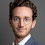## Thursday, March 17, 2011

### Lesson 29: General Class Exam Course G8B

Hello again!  The majority of lesson 29 deals with things we've covered in previous lessons.  The majority of this section deals with mixer and multiplier stages, deviation, and bandwidth.  There is also a little on data modes as well.

Mixers are stages in transmitters and receivers which combine two frequencies to produce a third.  This process is called heterodyning (remember the super heterodyne receivers we talked about in previous lessons).  When two signals are combined in a mixer, the new frequency will either be the sum or the difference of the two combined frequencies.  For instance, if a 10 MHz signal is received by an antenna is sent to a mixer and combined with an 8 MHz signal produced by an oscillator, the result will be an intermediate frequency (IF) of either 18 MHz or 2 MHz (10 MHz + 8 MHz or 10 MHz - 8 MHz).  The process to determine whether the IF is the sum or difference is fairly complicated and not important for the purposes of passing the exam.  The important things to know about mixers are they combine, or mix, two different frequency to produce a new frequency for the purposes of producing an IF or a desired transmitting frequency.

Multipliers perform a similar function as mixers in that they take an input signal and produce a new signal at a different frequency.  The difference is that a multiplier produces the new frequency by generating the harmonic of the input frequency.  This new frequency is usually double the input frequency.  For instance, if a 10 MHz signal is sent to the multiplier, the output frequency will be 20 MHz.  Multipliers are often used in VHF FM transmitters to change a modulated HF signal to a frequency in the VHF range.  These signals are often sent through several multiplier stages, doubling the frequency each time, until the desired transmitting frequency is reached.  The key word to remember when dealing with multipliers is "harmonic".

Another concept which may require some explanation is deviation.  Deviation primarily deals with FM signals.  A very simple explanation of deviation is that it is the difference between a carrier signal and frequency modulated signal, or the frequency differences between the modulated signal and the original carrier wave.  Deviation is expressed in terms of frequency (kHz, MHz, etc.).  For this section of questions, the thing to remember is that when a FM signal is sent through a multiplier, there is more or less a direct relationship between the signal frequency and the deviation.  If the frequency doubles, so does the deviation.

There is also a bandwidth formula you will need to memorize.  The formula is very simple.  If the deviation and modulated frequency are known, the formula is:

Bandwidth = 2(deviation + modulated frequency)

Easy enough.

Until the next lesson...

73,
Andy
KE4GKP

1.2.